Home > CC2 > Chapter 8 > Lesson 8.3.1 > Problem8-60

8-60.
1. Estimate the measure of each angle below. Homework Help ✎

1.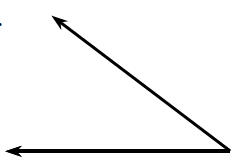2.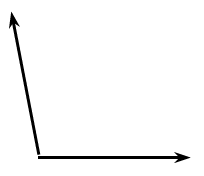3.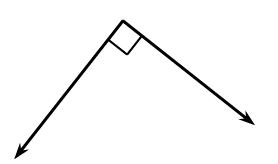4.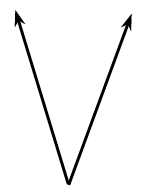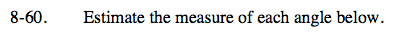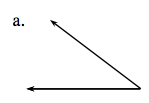The corner of your book is a right angle. Do you think this angle is bigger or smaller than the angle at the corner of your book? About how many of these angles could you put in the corner of your book?

This angle is approximately 40°.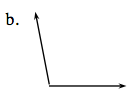Do you think this angle is bigger than the angle at the corner of your book? About how much bigger is it?

This angle is approximately 100°.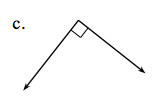A square in an angle means that the angle is 90°.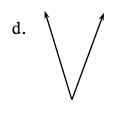How does this angle compare to the angles in parts(a), (b), and (c)? If it is bigger, how much bigger? If it is smaller, how much smaller?